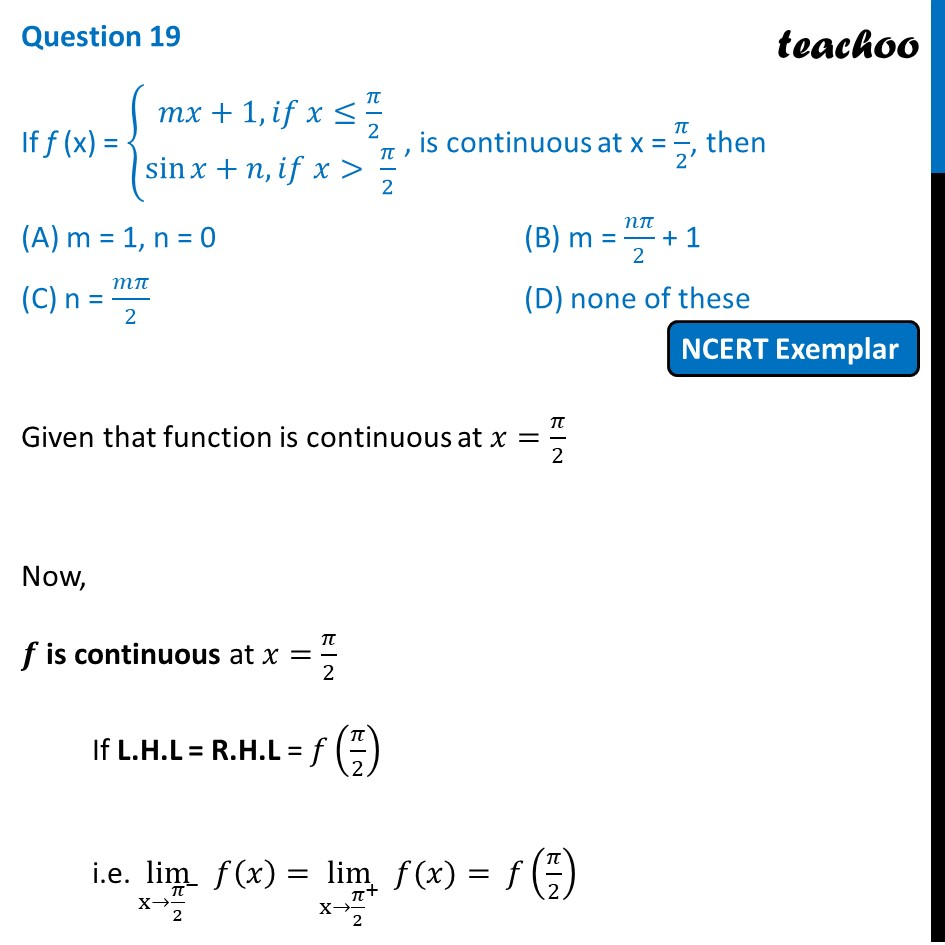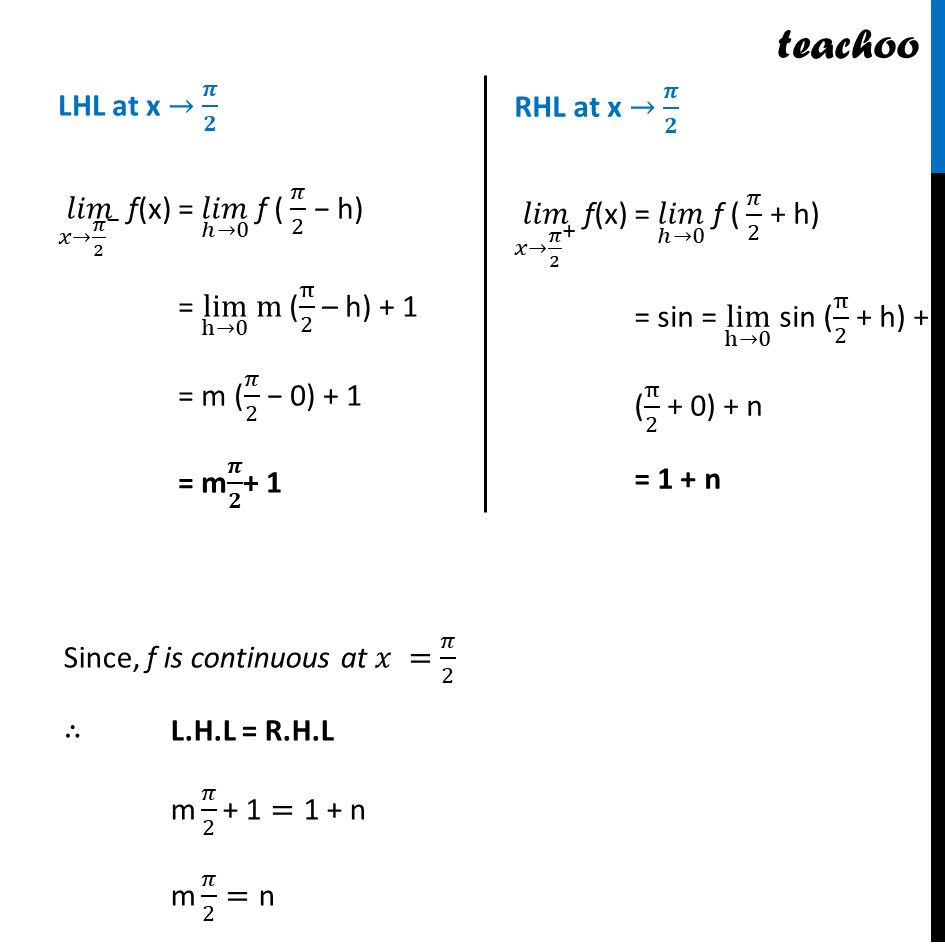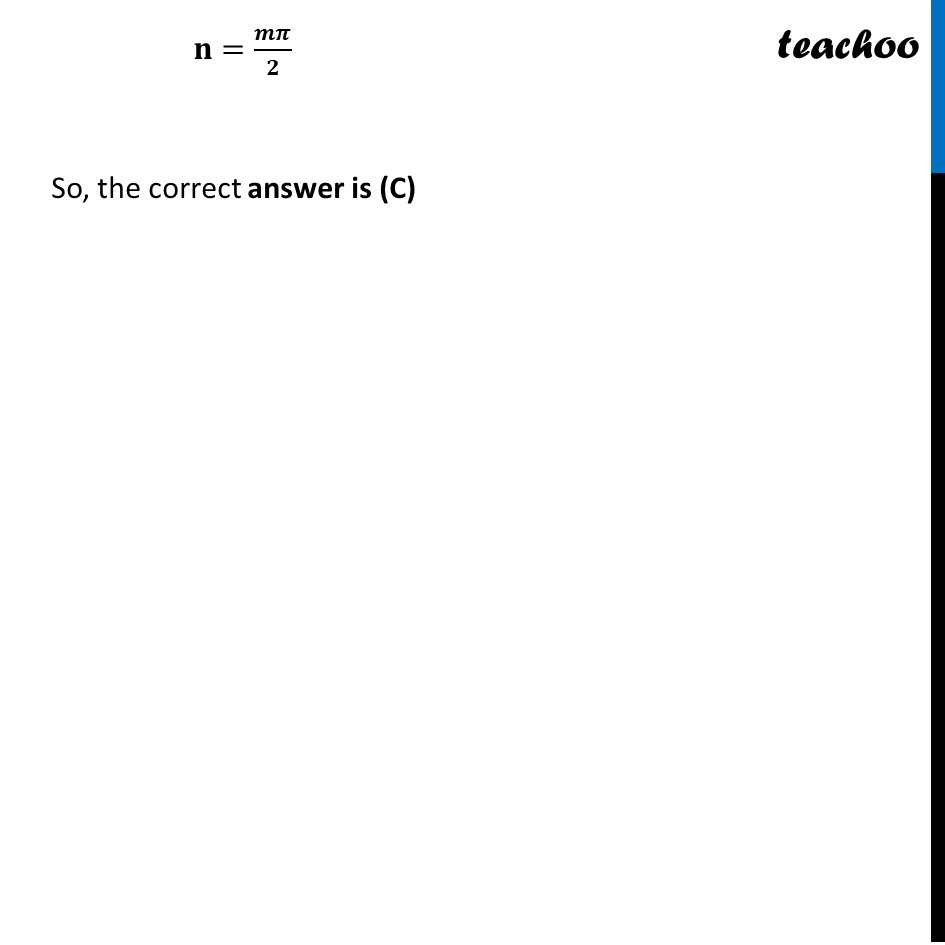NCERT Exemplar - MCQs

Chapter 5 Class 12 Continuity and Differentiability
Serial order wise

## (D) none of these

This question is similar to Ex 5.1, 28 - Chapter 5 Class 12 - Continuity and DifferentiabilityLearn in your speed, with individual attention - Teachoo Maths 1-on-1 Class

### Transcript

Question 17 If f (x) = {█(𝑚𝑥+1, 𝑖𝑓 𝑥≤𝜋/2@sin⁡〖𝑥+𝑛, 𝑖𝑓 𝑥> 𝜋/2〗 )┤ , is continuous at x = 𝜋/2, then (A) m = 1, n = 0 (B) m = 𝑛𝜋/2 + 1 (C) n = 𝑚𝜋/2 (D) none of these Given that function is continuous at 𝑥=𝜋/2 Now, 𝒇 is continuous at 𝑥=𝜋/2 If L.H.L = R.H.L = 𝑓(𝜋/2) i.e. lim┬(x→〖𝜋/2〗^− ) 𝑓(𝑥)=lim┬(x→〖𝜋/2〗^+ ) " " 𝑓(𝑥)= 𝑓(𝜋/2) LHL at x → 𝝅/𝟐 (𝑙𝑖𝑚)┬(𝑥→〖𝜋/2〗^− ) f(x) = (𝑙𝑖𝑚)┬(ℎ→0) f ( 𝜋/2 − h) = lim┬(h→0) m (π/2 – h) + 1 = m (𝜋/2 − 0) + 1 = m𝝅/𝟐+ 1 RHL at x → 𝝅/𝟐 (𝑙𝑖𝑚)┬(𝑥→〖𝜋/2〗^+ ) f(x) = (𝑙𝑖𝑚)┬(ℎ→0) f ( 𝜋/2 + h) = sin = lim┬(h→0) sin (π/2 + h) + n (π/2 + 0) + n = 1 + n Since, f is continuous at 𝑥 =𝜋/2 ∴ L.H.L = R.H.L "m" 𝜋/2 "+ 1"="1 + n" "m" 𝜋/2="n" 𝐧=𝒎𝝅/𝟐 So, the correct answer is (C)